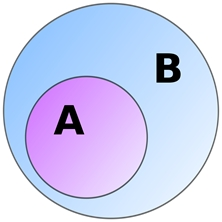# issubset()-in-python

PythonServer Side ProgrammingProgramming

#### Beyond Basic Programming - Intermediate Python

Most Popular

36 Lectures 3 hours

#### Practical Machine Learning using Python

Best Seller

91 Lectures 23.5 hours

#### Practical Data Science using Python

22 Lectures 6 hours

In this article, we will learn the implementation and usage of issubset() function available in the Python Standard LIbrary.

The issubset() method returns boolean True when all elements of a set are present in another set (passed as an argument) otherwise, it returns boolean False.

In the figure given below B is a subset of A. In case A & B are identical sets mean that A is a proper subset of B. This means both sets contain the same elements in them.## Syntax

<set 1>.issubset(<set 2>)

## Return value

boolean True/False

Now let’s look at an illustration to understand the concept.

## Example

A = {'t','u','t','o','r','i','a','l'}
B = {'t','u','t'}
C = {'p','o','i','n','t'}

print(B.issubset(A))
print(B.issubset(C))

A=set(str(A)+str(C))
print(C.issubset(A))

## Output

True
False
True

## Explanation

Here a check is made that all elements of B are contained in A which evaluates to be true. Similarly for the next statement output is produced.

Now we concatenated to sets to make it a subset forcefully by using typecasting as seen in the next statement.

Now let’s see what happens if we specify another type of iterable than set and pass it as an argument.

## Example

A = ['t','u','t','o','r','i','a','l']
B = {'t','u','t'}
C = ('p','o','i','n','t')
D = {'p','o','i','n','t'}

print(B.issubset(A))
print(B.issubset(C))

A=set(str(A)+str(C))
print(D.issubset(A))

## Output

True
False
True

## Explanation

Here we passed tuple, string and list iterables to the issubset() function. These types are implicitly converted to set type so as to get the desired output.

We must also observe that the argument outside the function must always of type <set> so that interpreter must know that comparison is between two sets and not any another type.

## Conclusion

In this article, we learnt how to use isubset() function in Python and what all types of arguments are allowed to be compared by the help of this function.